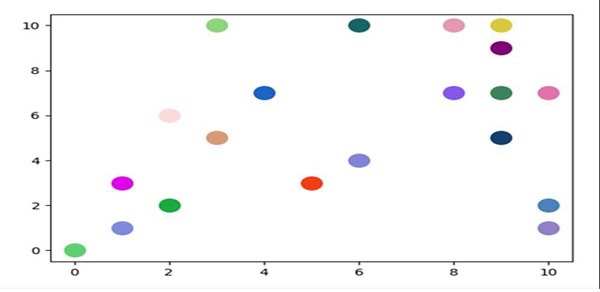# How to generate random colors in Matplotlib?

To make a custom color, we can create a hexadecimal string. From it, we can make different sets of color representation and can pass into the scatter method to get the desired output.

## Steps

• Take an input from the user for the number of colors, i.e., number_of_colors = 20.

• Use Hexadecimal alphabets to get a color.

• Create a color from (step 2) by choosing a random character from step 2 data.

• Plot scatter points for step 1 input data, with step 3 colors.

• To show the figure, use plt.show() method.

## Example

import matplotlib.pyplot as plt
import random

number_of_colors = int(input("Please enter number of colors: "))

color = ["#" + ''.join([random.choice(hexadecimal_alphabets) for j in
range(6)]) for i in range(number_of_colors)]

for i in range(number_of_colors):
plt.scatter(random.randint(0, 10), random.randint(0, 10), c=color[i], s=200)

plt.show()

## Output

Please enter the number of colors: 20Updated on: 16-Mar-2021

1K+ Views